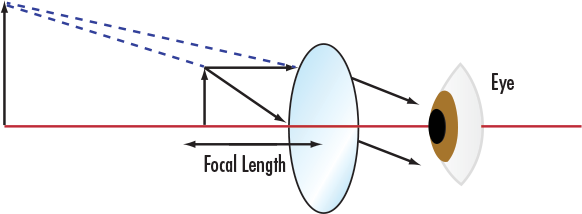# Simple Magnifier Calculator

View All Technical Tools

Magnifying Power, MP:

Focal Length (mm): --

Diopters (1/m): --$$\text{FL} = \frac{250 \text{mm}}{\text{MP}}$$
 $$\text{Diopters} = \frac{1000 \text{mm}}{\text{FL}}$$
 MP Magnifying Power
 FL Focal Length

## Description

Relate user supplied magnifying power to focal length and diopters of a lens. Calculator assumes 250mm as the near point of the human eye, closest distance the eye can focus for comfortable vision, in order to determine basic parameters for finding the best lens for one's desired magnification.

View All Now